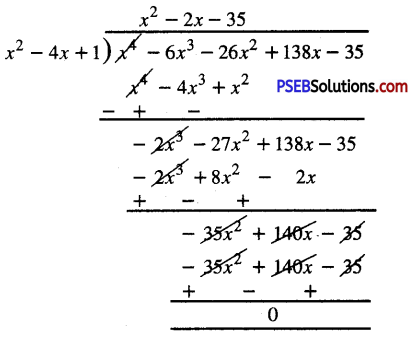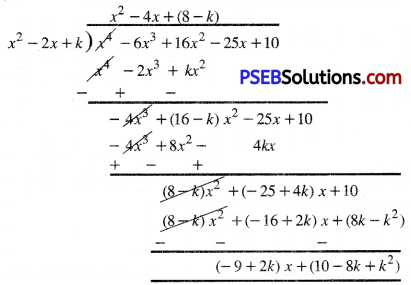# PSEB 10th Class Maths Solutions Chapter 2 Polynomials Ex 2.4

Punjab State Board PSEB 10th Class Maths Book Solutions Chapter 2 Polynomials Ex 2.4 Textbook Exercise Questions and Answers.

## PSEB Solutions for Class 10 Maths Chapter 2 Polynomials Ex 2.4

Question 1.
Verify that the number given alongside of the cubic polynomials below are their zeroes. Also verify the relationship between the zeroes and the coefficients in each case :
(i) 2x3 + x2 – 5x + 2; $$\frac{1}{2}$$, 1, -2
(ii) x3 – 4x2 + 5x – 2; 2, 1, 1
Solution:
(i) Let p (x) = 2x3 + x2 – 5x + 2
Compare it with ax3 + bx2 + cx + d
a = 2, b = 1, c = -5, d = 2
Now, P($$\frac{1}{2}$$) = 2$$\left(\frac{1}{2}\right)^{3}$$ + $$\left(\frac{1}{2}\right)^{2}$$ – 5$$\frac{1}{2}$$ + 2

= $$\frac{1}{4}+\frac{1}{4}-\frac{5}{2}$$

= $$\frac{1+1-10+8}{4}=\frac{0}{4}$$ = 0
∴ $$\frac{1}{2}$$ is zero of p (x)
and p(1) = 2(1)3 + (1)2 – 5 (1) + 2
= 2 + 1 – 5 + 2 = 5 – 5 = 0
∴ 1 is zero of p (x).
Also, p(-2) = 2(-2)3 + (- 2)2 -5(-2) + 2
= -16 + 4 + 10 + 2 = – 16+ 16 = 0
∴ – 2 is zero of p (x).
From above discussion, it is clear that $$\frac{1}{2}$$, 1, – 2 are the zeroes of given polynomial.
Let these zeroes are
α = $$\frac{1}{2}$$, β = 1, γ = – 2
Now, α + β + γ = $$\frac{1}{2}$$ + 1 + (-2)
= $$\frac{1}{2}$$ + 1 – 2
= $$\frac{1+2-4}{2}$$
= –$$\frac{1}{2}$$ = –$$\frac{b}{a}$$αβ + βγ + γα = ($$\frac{1}{2}$$) (1) + (-1) (-2) + (-2) ($$\frac{1}{2}$$)
= $$\frac{1}{2}$$ – 2 – 1
= $$\frac{1-4-2}{2}$$
= $$-\frac{5}{2}$$ = $$\frac{c}{a}$$

αβγ = ($$\frac{1}{2}$$) (1) (-2)
= ($$-\frac{2}{2}$$)
= ($$-\frac{d}{a}$$)
From above discussion, it is clear that there is a relationship between zeroes and coefficients.

(ii) Let p (x) = x3 – 4x2 + 5x – 2.
Compare it with ax3 + bx2 + cx + d
∴ a = 1, b = – 4, c = 5, d = – 2
Now p(2) = (2)3 – 4 (2)2 + 5 (2) – 2
= 8 – 16 + 10 – 2
= 18 – 18 = 0 2 is zero of p (2).
and p( 1) = (1)3 – 4(1)2 + 5(1) – 2
= 1 – 4 + 5 – 2 = 6 – 6 = 0
From above discussion it is clear that 2, 1, 1 are the zeroes of given polynomial. Ans.
Let these zeroes are
α = 2, β = 1, γ = 1
Now, α + β + γ = 2 + 1 + 1 = 4
= $$\frac{-(-4)}{1 \cdot}=\frac{-b}{a}$$
αβ + βγ + γα = (2) (1) + (1) (1) + (1) (2) = 2 + 1 + 2 = 5
= $$\frac{5}{1}=\frac{c}{a}$$

αβγ = (2) (1) (1) = 2
= $$\frac{-(-2)}{1}=\frac{-d}{a}$$
From above discussion, it is clear that there is a relationship between zeroes and coefficient.Question 2.
Find a cubic polynomial with the sum, sum of the product of its zeroes taken two at a time, and the product of its zeroes as 2, – 7, – 14 respectively.
Solution:
The general expression of cubic polynomial are
ax3 + bx2 + cx + d.
Let α, β, γ be its zeroes
α + β + γ = Sum of zeroes = 2
αβ + βγ + γα = Sum of product of zeroes = – 7
αβγ = Product of zeroes = – 14
∴ ax3 + bx2 + cx + d
= k [(x – α) (x – β) (x – γ)] where k is any constant.
= k [x3 – (α + β + γ) x2 + (αβ + βγ + γα)x – αβγ]
= k [x3 – 2x2 – 7x + 14] [Using (1)]
For different values of k, we get different cubic polynomial.Question 3.
If the zeroes of the polynomial x3 – 3x2 + x +1 are a – b, a, a + b, find a and b.
Solution:
Let p (x) = x3 – 3x2 + x + 1 and its zeroes are a – b, a, a + b.
a – b is zero of p (x) ………(Given)
∴ p (a – b) = 0
or (a – b)3 – 3 (a – b)2 + (a – b) + 1 = 0 or
[a3 – b3 – 3a2b + 3ab2] – 3 [a2 + b2 – 2ab] + a – b + 1 = 0
or a3 – b3 – 3a2b + 3ab2 – 3a2 – 3b2
+ 6ab + a – b + 1 = 0 ….(1)
and a is zero of p (x) …………(Given)
∴ p (a) = 0
or a3 – 3a3 + a + 1 = 0 ………….(2)
Also, a + b is zero of p (x) …(Given)
∴ p (a + b) = 0
or (a + b)3 – 3 (a + b)2 + (a + b) + 1 = 0
or (a3 + b3 + 3a2b + 3ab2) – 3 (a2 + b3 + 2ab) + a + b – 1 = 0 or
a3 + b3 + 3a2b + 3ab2 – 3a2 – 3b3 – 6ab + a + b + 1 = 0 …………….(3)
Adding (1) and (3), we get :
2a3 + 6ab2 – 6a2 – 6b2 + 2a + 2 = 0 or
a3+ 3ab2 – 3a2 – 3b2 + a + 1 = 0 or
(a3 – 3a3 + a + 1) + (3ab2 – 3b2) = 0 or
0 + 3b2 (a – 1) = 0 [Using (2)]
or a – 1 = 0
or a = 1 ………(4)
From (3) and (4), we get:
(1)3 + b3 + 3(1)2b + 3(1 )b2 – 3(1)2 – 3b2 – 6 (1) b + 1 + b + 1 = 0 or
1 + b3+ 3b + 3b2 – 3 – 3b2 – 6b + b + 2 = 0
or b3 – 2b = 0 or
b (b2 – 2) = 0 or
b2 – 2 = 0 or
b2 = 2
or b = ±√2
Hence a = 1, b = ±√2Another Solution:
Given that three zeroes of polynomial x3 – 3x2 + x + 1 are a – b, a, a + b respectively
Now, sum of zeroes = (a – b) + a + (a + b) = a – b + a + a + b = 3a
But, sum of zeroes using coefficient = $$\frac{-\text { Coefficient of } x^{2}}{\text { Coefficient of } x^{3}}$$
= $$\frac{-(-3)}{1}$$ = 3
∴ 3a = 3 or a = 1
Also, product of zeroes = (a – b) . a . (a + b) = (a2 – b2) a
Putting the value of a, we get :
= (12 – b2) . 1
= 1 – b2
But, product of zeroes using coefficient = $$=\frac{-\text { Constant term }}{\text { Coefficient of } x^{3}}$$
= $$\frac{-1}{1}$$ = -1
1 – b2 = – 1
– b2 = – 1 – 1
– b2 = – 2 or
b2 = 2
b = ±√2
Hence a = 1 and b = ±√2

Question 4.
If two zeroes of the polynomial x4 – 6x3 – 26x2 + 138x – 35 are 2 ± √3, find other zeroes.
Solution:
Given that two zeroes are (2 + √3) and (2 – √3)
∴ [x – (2 + √3)] [x – (2 – √3)] are factors of given polynomial.
Now, [x – (2 + √3)] [x – (2 – √3)]
= x2 – [2 – √3 + 2 + √3]x + [(2 + √3) + (2 – √3)]
= x2 – 4x + [(2)2 – (√3)2]
= x2 – 4x + 1
∴ (x2 – 4x + 1) is factor of given polynomial. Now, apply division algorithm to given polynomial and (x2 – 4x + 1)∴ x4 – 6x3 – 26x2 + 138x – 35
= (x2 – 4x + 1) (x2 – 2x – 35)
= (x2 – 4x + 1) [x2 + 5x – 7x – 35]
S = – 2, P = – 35
= (x2 – 4x + 1) [x (x + 5) – 7 (x + 5)]
= (x2 – 4x + 1) (x + 5).(x – 7)
Now, other zeroes of polynomials are given by :
x + 5 = 0 Or x – 7 = 0
x = – 5 Or x = 7
∴ the zeroes of the given fourth degree polynomial are :
2 + √3, 2 – √3, -5, 7.Question 5.
If the polynomial x4 – 6x3 + 16x2 – 25x + 10 is divided by another polynomial x2 – 2x + k, the remainder comes out to be x + a, find k and a.
Solution:
Given that, polynomial x4 – 6x3 + 16x2 – 25x + 10 is divided by another polynomial x2 – 2x + k then remainder comes out to be x + a
So, first of all we divide,
x4 – 6x3 + 16x2 – 25x + 10 with x2 – 2x + k and find quotient and remainder.∴ by division algorithm for Polynomials x4 – 6x3 + 16x2 – 25x +10
= (x2 – 2x + k) [x2 – 4x + (8 – k) + [(- 9 + 2k) x + (10 – 8k + k2]
Quotient = x2 – 4x + (8 – k) and
Remainder = (- 9 + 2k) x + (10 – 8k + k2)
But, remainder = x + a ….(Given)
∴ (- 9 + 2k) x + (10 -8k + k2) = x + a
Compare the like coefficients, we get :
-9 + 2k = 1 Or
2k = 1 + 9
2k = 10
k = $$\frac{10}{2}$$ = 5 or

10 – 8k + k2 = a
Putting the value of k, we get
10 – 8 × 5 + (5)2 = a
10 – 40 + 25 = a
-5 = a
a = -5
Hence k = 5 and a = -5